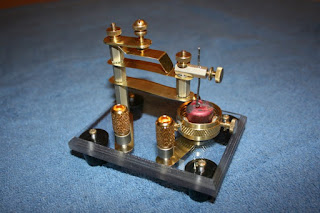## Thursday, July 2, 2015

### Atomic Lattices and Glowscript

I'm finishing up (read: procrastinating finishing up) a final paper in a material science course that I'm taking this summer. The paper is about cuprous oxide ($Cu_2O$) and its possible use in a homemade diode. That process is outlined here.HP Friedrichs's homemade diode and holder
I am investigating whether what he has described in terms of process and results jibes with the literature's descriptions of fabrication processes, physical properties, and and electrical properties. All of that's interesting, but one neat part was a look at the crystal structure. It's a combination of two crystals: the copper forms a face-centered cubic sublattice and the oxygen forms a body-centered cubic sublattice.

Specifying crystals, I've learned, is a pretty neat vector operation, and one which lends itself to programming pretty well. There's a basic unit which is repeated at each point of a cube. That unit isn't necessarily as many atoms as you might think: for a body-centered cubic lattice, it's a set of points at (relative coordinates) (0,0,0) and a(.5,.5,.5), where a is the lattice constant, which is the edge length of the cube. hen you replicate this two-atoms basis at each of the corners of a cube of side-length a, you get a body-centered cubic lattice (doing it just once gives some extra atoms; the unit cell consists of just those atoms within the unit cube. Doing it infinitely, though, will give an infinite BCC lattice). It's a little harder to picture the FCC lattice, but its basis consists of (0,0,0), a(0,.5,.5), a(.5,0,.5), and a(.5,.5,0).

So we have a set of atom positions (2 or 4, depending on the lattice) that we want to iterate over all point of the form a(x, y, z), with all coordinates in the integers. That's a perfect setup for VPython/Glowscript. It's easy enough, using some for loops, to iterate over an area of desired dimensions, and then it can all be zoomed and rotated by the user with VPython/Gloscript's native controls. I added a box showing the unit cube for orientation, and.. voila (click image or here to see animation)!GlowScript 1.1 VPython

scene.background = color.white

# apply basis atoms to each site in cubic lattice
atoms = []
for site in cubic:
for atom in basis:
atoms.append(sphere(color= color, radius = rad, pos = site+atom+offset))
return atoms

# apply basis atoms to each site in cubic lattice
atoms = []
for site in cubic:
for atom in basis:
if 0 <= (site+atom+offset).x <= a and 0 <= (site+atom+offset).y <= a and 0 <= (site+atom+offset).z <= a:
atoms.append(sphere(color= color, radius = rad, pos = site+atom+offset))
return atoms

def cubeDraw(color):
# draw lines around the unit (not primitive) cell
curve(pos = [a*vector(0,0,0),a*vector(1,0,0),a*vector(1,1,0),a*vector(0,1,0),a*vector(0,0,0)], color = color)
curve(pos = [a*vector(0,0,0),a*vector(0,0,1),a*vector(0,1,1),a*vector(0,1,0),a*vector(0,0,0)], color = color)
curve(pos = [a*vector(0,0,1),a*vector(1,0,1),a*vector(1,1,1),a*vector(0,1,1),a*vector(0,0,1)], color = color)
curve(pos = [a*vector(1,0,0),a*vector(1,0,1),a*vector(1,1,1),a*vector(1,1,0),a*vector(1,0,0)], color = color)

a = 1 # lattice constant

# Lattice maker
# basis vectors
bcc = [vector(0,0,0), vector(.5*a,.5*a,.5*a)]
fcc = [vector(0,0,0), vector(0,.5*a,.5*a), vector(.5*a,0,.5*a), vector(.5*a,.5*a,0)]

# Create unit cubic lattice
# horizontal extent (will go from -x to x)
xmin = -1.5
xmax = 1.5
# vertical extent (will go from -y to y)
ymin = -1.5
ymax = 1.5
#in-out extent (will go from -z to z)
zmin = -1.5
zmax = 1.5

cubic = []

for i in arange(xmin,xmax+1,1):
for j in arange(ymin,ymax+1,1):
for k in arange(zmin,zmax+1,1):
cubic.append(a*vector(i,j,k))

# Use this to show a single unit cell
#CuAtoms = makeCellLattice(cubic,bcc,vector(0,0,0),color.red,a/15)
#OAtoms = makeCellLattice(cubic,fcc,(sqrt(3)/8)*a*vector(-1,-1,-1),color.blue,a/20)

#Use this to show a bigger lattice
CuAtoms = makeLattice(cubic,bcc,vector(0,0,0),color.red,a/15)
OAtoms = makeLattice(cubic,fcc,(sqrt(3)/8)*a*vector(-1,-1,-1),color.blue,a/20)

scene.center = a*vector(.5,.5,-.5)

cubeDraw(color.red)